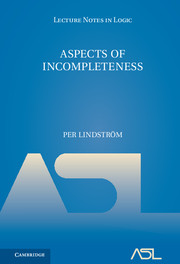# Lecture Notes in Logic, 10

## Aspects of Incompleteness

Per LindströmYear: 2017
ISBN: 9781107167926

In this volume, the tenth publication in the Lecture Notes in Logic series, Per Lindström presents some of the main topics and results in general metamathematics. In addition to standard results of Gödel et al. on incompleteness, (non-)finite axiomatizability, and interpretability, this book contains a thorough treatment of partial conservativity and degrees of interpretability. It comes complete with exercises, and will be useful as a textbook for graduate students with a background in logic, as well as a valuable resource for researchers.

• Preface
• Introduction
1. Preliminaries
2. Incompleteness
3. Numerations of r.e. sets
4. Axiomatizations
5. Partial conservativity
6. Interpretability
7. Degrees of interpretabilty
8. Generations
• References
• Index
• Notation